# Rotation Of Complex Numbers

Rotation is a convenient method that is used to relate complex numbers and angles that they make; this method will be widely used subsequently. However, you will realize that the method involves no new concept.

Consider a configuration of complex numbers as shown below: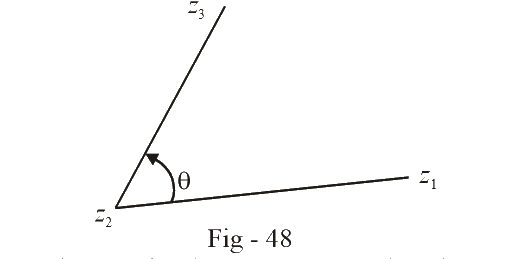We know the angle $$\theta$$. Our purpose is to write down an expression that relates all the four quantities $${z_1},\,\,{z_2},\,\,{z_3}$$ and $$\theta$$.

Consider the vector $${z_3} - {z_2}.$$  Let its argument be $${\theta _1}.$$ Similarly, let the argument of the vector $${z_1} - {z_2}$$  be $${\theta _2}.$$ Now, a little thought will show you that $$\theta$$ is simply $${\theta _1} - {\theta _2}$$.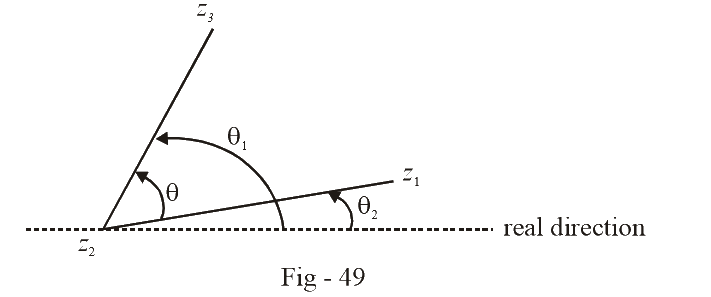Now we write $${z_3} - {z_2}\;\;and\;\;\;{z_1} - {z_2}$$ in Euler’s form

$\begin{array}{l}{z_3} - {z_2} = \left| {{z_3} - {z_2}} \right|{e^{i{\theta _1}}}\,\,\,\,\,\,\,\,\,\,\,\,\,\,\,\,\,...{\rm{ }}\left( 1 \right)\\{z_1} - {z_2} = \left| {{z_1} - {z_2}} \right|{e^{i{\theta _2}}}\,\,\,\,\,\,\,\,\,\,\,\,\,\,\,\,...{\rm{ }}\left( 2 \right)\end{array}$

Since we know  $${\theta _1} - {\theta _2}$$, we divide (1) by (2) to get

$\frac{{{z_3} - {z_2}}}{{{z_1} - {z_2}}} = \frac{{\left| {{z_3} - {z_2}} \right|}}{{\left| {{z_1} - {z_2}} \right|}}\,\,{e^{i\theta }}\,\,\,\,\,\,\,\,\,\,\,\,\,\,\,\,...{\rm{ }}\left( 2 \right)$

This is the relation we were looking for. It relates all the four terms $${z_1},{z_2},{z_3}\;\,{\rm{and}}\,\,\theta$$.

You might have wondered why this method is called rotation. Well, you can think of this method in this way: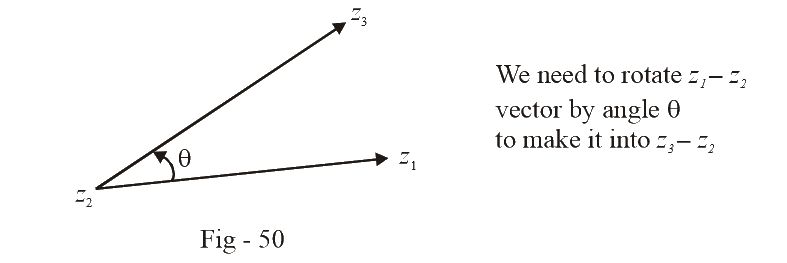You are given the vector $${z_1} - {z_2}$$ . You need to modify it into the vector $${z_3} - {z_2}.$$ How can you do it? Obviously, there will be a change in modulus. Apart from that, you need to rotate the vector $${z_1} - {z_2}$$  anticlockwise by angle $$\theta$$ too. This is where the term rotation comes from. Viewing the process in this way we obtain the relation (3) as follows

Write down the unit vector in the direction of the original vector, the one that you need to rotate. In our case, it will be

\begin{align}&\frac{{{z_1} - {z_2}}}{{\left| {{z_1} - {z_2}} \right|}}\end{align}

To rotate this unit vector by an angle $$\theta$$  (anticlockwise; for clockwise, it will be – $$\theta$$  ), we multiply it by $${e^{i\theta }}$$   . For the current case, this turns the unit vector into a new unit vector along the direction of the vector $${z_3} - {z_2}:$$

    $\frac{{{z_1} - {z_2}}}{{\left| {{z_1} - {z_2}} \right|}}\,{e^{i\theta }}$

Finally, to turn this unit vector into the final vector that we need to obtain after rotation, we multiply the unit vector by the appropriate magnitude. Thus we obtain the required final vector after rotation

\begin{align}&{z_3} - {z_2} = \left| {{z_3} - {z_2}} \right| \cdot \frac{{{z_1} - {z_2}}}{{\left| {{z_1} - {z_2}} \right|}}{e^{i\theta }}\end{align}

This is the same relation that we obtained in (3)

Using this same approach, suppose that we now wish to relate four complex numbers in the following configuration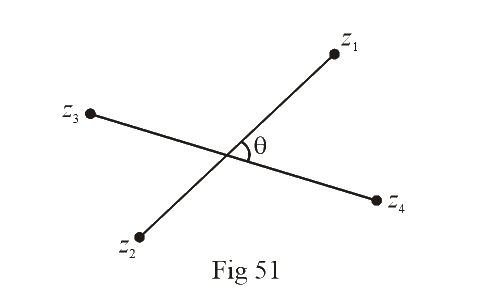Using rotation, we can obtain the vector $${z_1} - {z_2}$$  from the vector $${z_4} - {z_3}:$$

Unit vector along

$${z_4} - {z_3}$$ direction : \begin{align}&\frac{{{z_4} - {z_3}}}{{\left| {{z_4} - {z_3}} \right|}}\end{align}

(Initial unit vector)

Unit vector along

$${z_1} - {z_2}$$  direction   :  \begin{align}&\frac{{{z_1} - {z_2}}}{{\left| {{z_1} - {z_2}} \right|}}\end{align}

(Final unit vector)

$$\text{Angle between the two }\;\;:\;\;\;\quad\theta$$

\begin{align}&\Rightarrow \frac{{{z_1} - {z_2}}}{{\left| {{z_1} - {z_2}} \right|}} = \frac{{{z_4} - {z_3}}}{{\left| {{z_4} - {z_3}} \right|}}\,\,{e^{i\theta }}\end{align}

This is the relation we wished to obtain.

Example-  21

Prove that if the complex numbers $${z_1},\,\,{z_2}$$  and the origin form an equilateral triangle, then $$z_1^2 + z_2^2 - {z_1}{z_2} = 0$$ .

Solution: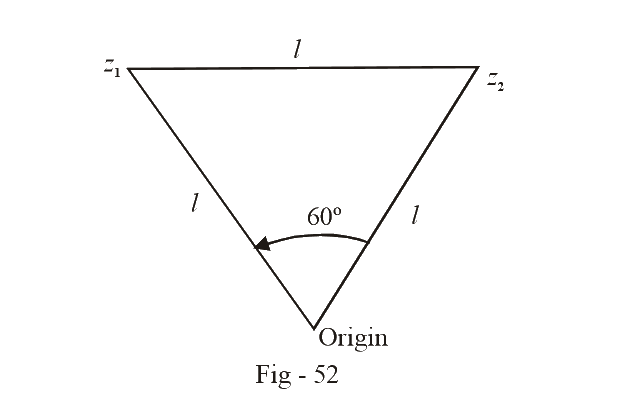We can assume the configuration drawn above for $${z_1}$$ and $${z_2}.$$ Observe that the vector $${z_1}$$  can be obtained from the vector $${z_2}$$  through rotation.

\begin{align}&\,\,\,\,\,\,\,\,\frac{{{z_1}}}{l} = \frac{{{z_2}}}{l}{e^{i\pi /3}}\\ &\Rightarrow \frac{{{z_1}}}{{{z_2}}} = {e^{i\pi /3}} = \cos \frac{\pi }{3} + i\sin \frac{\pi }{3}\\\,\,\,\,\,\,\,\,\,\,\,\, &\qquad\qquad\qquad= \frac{1}{2} + i\frac{{\sqrt 3 }}{2}\\&\Rightarrow \frac{{z_1^2}}{{z_2^2}} = \frac{1}{4} - \frac{3}{4} + \frac{1}{2}i\,\sqrt 3 \\\,\,\,\,\,\,\,\,\,\,\,\, &\qquad\quad= - \frac{1}{2} + i\frac{{\sqrt 3 }}{2}\\\,\,\,\,\,\,\,\,\,\,\, &\qquad\quad= - 1 + \left( {\frac{1}{2} + i\frac{{\sqrt 3 }}{2}} \right)\\\,\,\,\,\,\,\,\,\,\, &\quad\quad\quad= - 1 + \frac{{{z_1}}}{{{z_2}}}\\&\Rightarrow z_1^2 = - z_2^2 + {z_1}{z_2}\\&\Rightarrow z_1^2 + z_2^2 - {z_1}{z_2} = 0\end{align}

Example- 22

Find the vertices of a regular polygon of n sides if its center is at the origin and one of its vertices is known to be   $${z_1}$$.

Solution: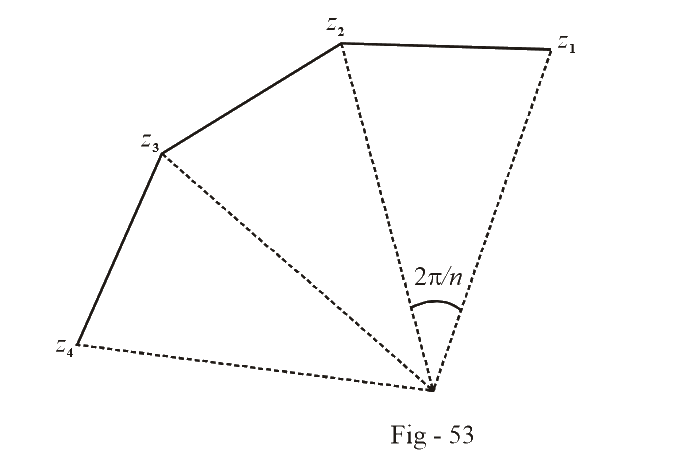From the figure, it should be clear that all the vertices of the polygon (other than $${z_1}$$) can be obtained from $${z_1}$$ through successive rotations, each of angle  $$2\pi /n$$.

\begin{align}&{z_2} = {z_1}{e^{i2\pi /n}}\\&{z_3} = {z_2}{e^{i2\pi /n}} = {z_1}{e^{i4\pi /n}}\\&{z_n} = {z_{n - 1}}{e^{i2\pi /n}} = {z_1}{e^{i2(n - 1)\pi /n}}\end{align}

If we let $${e^{i2\pi /n}}$$ be $$\beta$$, then

\begin{align}&{z_2} = \beta {z_1},{z_3} = {\beta ^2}{z_1}.........,{z_n} = {\beta ^{n - 1}}{z_1}.\end{align}

Thus, all the vertices become known.

Learn from the best math teachers and top your exams

• Live one on one classroom and doubt clearing
• Practice worksheets in and after class for conceptual clarity
• Personalized curriculum to keep up with school